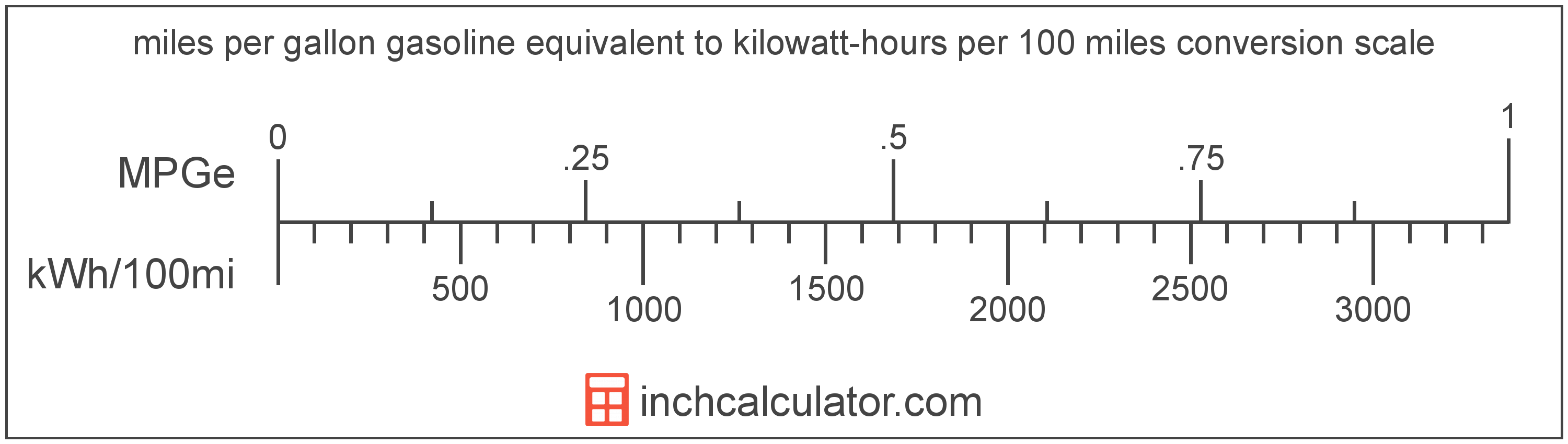# Miles per Gallon Gasoline Equivalent to Kilowatt-Hours per 100 Miles Converter (MPGe to KWh/100mi)

Enter the electric car efficiency in miles per gallon gasoline equivalent below to get the value converted to kilowatt-hours per 100 miles.

Results in Kilowatt-Hours per 100 Miles:1 MPGe = 3,370.5 kWh/100mi

Do you want to convert kWh/100mi to MPGe?

## How to Convert Miles per Gallon Gasoline Equivalent to Kilowatt-Hours per 100 Miles

Convert miles per gallon gasoline equivalent to kilowatt-hours per 100 miles with this simple formula:

kWh/100mi = 3370.5 ÷ MPGe

Insert the MPGe efficiency measurement in the formula and then solve to find the result.

For example, let's convert 50 MPGe to kWh/100mi:

50 MPGe = ( 3370.5 ÷ 50 ) = 67.41 kWh/100miMiles per gallon gasoline equivalent and kilowatt-hours per 100 miles are both units used to measure electric car efficiency. Keep reading to learn more about each unit of measure.

## What Are Miles per Gallon Gasoline Equivalent?

Miles per gallon gasoline equivalent is a way of measuring the energy needed equivalent to a gallon of gasoline to travel a distance in miles.

Because electric vehicles do not consume gasoline, the EPA set an equivalent amount of energy in kilowatt-hours that is equal to one gallon of gasoline. According to the EPA, one gallon of gasoline is equal to 33.705 kilowatt-hours.

The mile per gallon gasoline equivalent is a US customary unit of electric car efficiency. Miles per gallon gasoline equivalent can be abbreviated as MPGe; for example, 1 mile per gallon gasoline equivalent can be written as 1 MPGe.

## What Are Kilowatt-Hours per 100 Miles?

Kilowatt-hours per 100 miles are a measure of electric vehicle efficiency measuring the kilowatt-hours of energy required to travel 100 miles.

The kilowatt-hour per 100 miles is a US customary unit of electric car efficiency. Kilowatt-hours per 100 miles can be abbreviated as kWh/100mi; for example, 1 kilowatt-hour per 100 miles can be written as 1 kWh/100mi.

In the expressions of units, the slash, or solidus (/), is used to express a change in one or more units relative to a change in one or more other units.

## Mile per Gallon Gasoline Equivalent to Kilowatt-Hour per 100 Miles Conversion Table

Table showing various mile per gallon gasoline equivalent measurements converted to kilowatt-hours per 100 miles.
Miles Per Gallon Gasoline Equivalent Kilowatt-hours Per 100 Miles
0.001 MPGe 3,370,500 kWh/100mi
0.002 MPGe 1,685,250 kWh/100mi
0.003 MPGe 1,123,500 kWh/100mi
0.004 MPGe 842,625 kWh/100mi
0.005 MPGe 674,100 kWh/100mi
0.006 MPGe 561,750 kWh/100mi
0.007 MPGe 481,500 kWh/100mi
0.008 MPGe 421,313 kWh/100mi
0.009 MPGe 374,500 kWh/100mi
0.01 MPGe 337,050 kWh/100mi
0.02 MPGe 168,525 kWh/100mi
0.03 MPGe 112,350 kWh/100mi
0.04 MPGe 84,263 kWh/100mi
0.05 MPGe 67,410 kWh/100mi
0.06 MPGe 56,175 kWh/100mi
0.07 MPGe 48,150 kWh/100mi
0.08 MPGe 42,131 kWh/100mi
0.09 MPGe 37,450 kWh/100mi
0.1 MPGe 33,705 kWh/100mi
0.2 MPGe 16,853 kWh/100mi
0.3 MPGe 11,235 kWh/100mi
0.4 MPGe 8,426 kWh/100mi
0.5 MPGe 6,741 kWh/100mi
0.6 MPGe 5,618 kWh/100mi
0.7 MPGe 4,815 kWh/100mi
0.8 MPGe 4,213 kWh/100mi
0.9 MPGe 3,745 kWh/100mi
1 MPGe 3,371 kWh/100mi

## References

1. United States Environmental Protection Agency, Technology, https://www3.epa.gov/otaq/gvg/learn-more-technology.htm